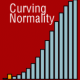R-Sessions 22: Book: Introductory Statistics with R — Peter Dalgaard (2002)

Introductory Statistics with RPeter Dalgaard is associate professor at the Department of Biostatistics at the University of Copenhagen in Denmark, and a member of the R-Project Core Development team. Also, he is an active participating and respected member of the R-help mailing-list. Based on these experiences, he set to write an introductory book on statistics and R.

The book start with relatively simple topics, easily working toward more complex statistical problems. Central techniques that are covered are analysis of variance and regression. Starting with bivariate analyses, multivariate analyses of both types are discussed to a high extent. Several types of linear (regression) models are introduced, covering polynomial regression, regression without an intercept, interactional model, two-way ANOVA with replication, and ANCOVA. A separate chapter focusses on logistic regression. Moreover, in many ways the equivalence or parallels of regression and ANOVA are discussed. Thereby, a greater understanding of the (differences between) techniques is stimulated.

In the course of the book, the reader is introduced to R by simple examples. The book has a R-package available for download that contains several data-sets that are used throughout the book. An appendix in the book describes the data-sets. In contrast with many other books on R, the package does not contain functions that were written by the author. The benefit of that is, is that the books only relies on the most basic functions that come with a basic installation of R. When the book was written, the most recent version of R was 1.5.0. Presently, it is 2.4.1, but all the examples still work without any problem.

The power of the approach in this book, is that both the statistical techniques are introduced, as well as it is shown how to perform these tests using R. But, while the book aims to be an introduction to statistics, it somewhat fails to introduce the techniques thoroughly. Strikingly, the author does not explain (much) on the interpretation of results. For instance, no guidance is given in the interpretation of the results of a logistics regression. This cannot be expected to be clear for a novice in statistics. If a reader does not have any understanding of statistics prior to reading this book, the book will fail in its’ purpose. To put it differently: the book hovers somewhere in between “Introductory Statistics with R” and “Introduction to R, using Statistics”.

Any author makes choices when writing, based on his or her background. Differences occur in the subjects that are addressed. In this, Peter Dalgaard has made some nice choices, that make to book pleasantly distinguishing from other introductory books. The cross-over between regression and ANOVA on the applied level has already been mentioned. Other niceties are a chapter dedicated to determining the statistical power of tests. To students starting to learn about statistics, this might be a starting point for discussing the relevancy of statistical significance. Another distinguishing chapter is a chapter on survival analysis. Not often found in starting books on statistics, this chapter shows that statistics in different contexts are based still on the same principles.

Nevertheless, the book seems ideal for teaching purposes in combination with a book that handles more fundamental issues of statistics. If used in that way, a powerful combination is at hand. The lack of guidance in interpretation then is not so much of a problem. A difficulty many students in statistics have, is transferring their new knowledge on a fundamental level to application. This book will be of help, mostly because it has an emphasis on application, but explains some of the more important fundamental issues, from a practical perspective. Thereby, when used with prior knowledge or an additional book on statistics, it is a wonderful addition to applied statistics and R-Project.

– – — — —– ——–

– – — — —– ——–
R-Sessions is a collection of manual chapters for R-Project, which are maintained on Curving Normality. All posts are linked to the chapters from the R-Project manual on this site. The manual is free to use, for it is paid by the advertisements, but please refer to it in your work inspired by it. Feedback and topic requests are highly appreciated.
——– —– — — – –

2 comment on “R-Sessions 22: Book: Introductory Statistics with R — Peter Dalgaard (2002)”

•meselu

•